Queries for Skew partitions: search statistic / browse statistics / browse maps from / browse maps to

# Definition & Example

• A skew partition $(\lambda,\mu)$ of $n \in \mathbb{N}_+$ is a pair of integer partitions such that $\mu \subseteq \lambda$ as Ferrers diagrams.

• Skew partitions are graphically represented by their Ferrers diagram (or Young diagram) as the collection of boxes of $\lambda$ that are not boxes of $\mu$.

• A skew partition is reduced if its Ferrers diagram does not contain empty rows before the last nonempty row and empty columns before the last nonempty column.

• We write $(\lambda,\mu) \vdash n$ if $\lambda$ is a partition of $n$.

 the 9 Skew partitions of size 3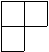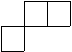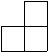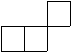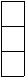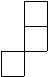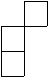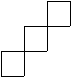[,[]] [[2,1],[]] [[3,1],] [[2,2],] [[3,2],] [[1,1,1],[]] [[2,2,1],[1,1]] [[2,1,1],] [[3,2,1],[2,1]]

• The number of skew partitions is A225114.

TBA

# Technical information for database usage

• A skew partition is uniquely represented as a list of pairs representing the two integer partitions.
• Skew partitions are graded by the size of the bigger partition minus the size of the smaller one.
• The database contains all integer partitions of size at most 7.

If you want to edit this wiki page, you can download the raw markdown and send your new version to info@findstat.org